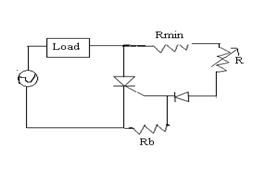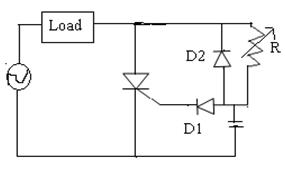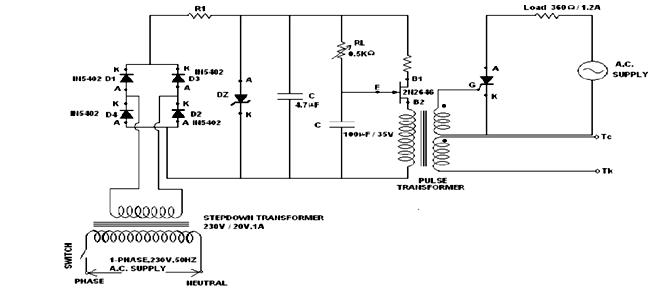# Power Control Using SCR

### SCR

SCR or Silicon Controlled Rectifier is a 3 pin device, having three basic terminals- anode, cathode and gate. The gate terminal is the control terminal for the application of anode-cathode voltage. Typically Silicon is used because of its low leakage current. The polarity of the voltages applied to the cathode and anode decides whether the device is in forward bias or reverse bias and the gate voltage decides the conduction of the SCR. In other words, when forward bias is applied to the SCR, after proper positive gate voltage is applied, the device starts conducting and is turned off only when the current through the device is made less than the holding current. Thus the SCR can be used as a switch.

### SCR Firing:

The application of GATE voltage is known as firing.

### Types of SCR firing:

Generally, there are two types of firing:

• Zero Voltage Cross Over firing: Zero-crossing control mode (also called fast cycling, integral cycle, or burst firing) operates by turning the SCR’s on only when the instantaneous value of the sinusoidal voltage is zero.
• Phase angle control method: The phase angle is varied, i.e. the application of gate pulses is delayed by a certain time and the conduction is controlled.

### Firing Circuits:

#### Features of the firing circuit:

• Firing circuits should produce trigger pulses for the thyristor at appropriate instants.
• There needs to be electrical isolation between firing circuits and the thyristor. It is achieved using a pulse amplifier or an optoisolator.

### Types of firing circuit:

• R-Firing circuit:• RC firing circuit:• UJT firing circuit:### Firing Angle:

The number of degrees from the beginning of the cycle when SCR is switched on is firing angle.  Any SCR would start conducting at a particular point on the ac source voltage. The particular point is defined as the firing angle. The earlier in the cycle the SCR is gated ON, the greater will be the voltage applied to the load.

### Firing Angle Control:

Firing angle control can be used in applications like controlling the speed of fan motors, controlling the intensity of a bulb, by controlling the application of power to the SCR. The firing angle control is achieved by varying the time of application of Gate pulses to the SCR. The voltage to the Gate terminal of the SCR can be applied at a given time decided by the remote input.

Basically by controlling the firing angle means managing the point on the AC signal waveform when the SCR is going to be triggered or in other words, the time corresponding to the AC signal waveform when the SCR gate is going to be given DC supply voltage. Normally to trigger an SCR we use optoisolator. For a simple power application circuit, where no power control is required, normally zero-crossing detectors or optoisolators having zero-crossing detectors can be used, by which the SCR is triggered only at the zero crossing levels of the AC waveform. For other applications involving power controlling application, the gate is triggered using pulses and the firing angle is varied accordingly to control the switching of the SCR and accordingly the power of the SCR.

The variation in firing angle or variation of conduction of SCR by delaying the application of gate current can be done in two ways:

• Phase Shifting Gate control: It causes 0 to 180⁰ delay of conduction. The phase angle of the gate voltage is changed with respect to the anode-cathode voltage. In other words, the gate voltage is applied out of phase with the anode voltage.

Usually, capacitance or inductances are used for this purpose. In the LR combination, the current lags the voltage, whereas, in an RC combination, currently leads the voltage. The resistor R is varied so as to vary the phase angle by which the gate voltage delays from the anode voltage.

Different circuits used as a phase shifter are as given:

• Pulse Triggering: The gate voltage can also be applied by giving pulses to the Gate terminal. The duty cycle of the pulses can be varied to provide variation in the conduction.

Pulses can be generated either using UJT or using 555 timers.

Working Example of Firing Angle control and its application

The above block diagram represents the system for achieving power control to induction motor using firing angle control for back to back SCRs.

Before going into details about how firing angle control is achieved in this system, let us have a quick look at back to back connection of SCR.

Here is a video describing a back to back SCR connection.

A back to back SCR connection is used to provide AC power to the load in both half cycles of the AC signal. Two optoisolators are connected to each SCR. In the first half cycle of the AC signal, one of the SCR conducts after getting triggered using an optoisolator and allows the current to pass through the load. In the second half cycle, another SCR connected in reverse direction to that of the other SCR, gets triggered using another optoisolator and allows current to flow to the load. Thus the load gets AC power in both the half cycles.

In this system, the SCR is triggered using an optoisolator containing a combination of a LED and a TRIAC. When pulses are applied to the LED, it emits light which falls on the TRIAC and it conducts, causing the output pulses from the optoisolator to the SCR. The principle involves controlling the rate of application of pulses by varying the frequency between adjacent pulses. A microcontroller is used to provide pulses to the optoisolator based on the push button input interfaced to it. The number of times the push button is pressed decides the amount of delay of application of pulses. For example, if the push button is pressed once, the Microcontroller delays the application of pulse by 1ms. Thus the angle at which the SCR is triggered is controlled accordingly and the application of AC power to the load is controlled.

Photo Credit: